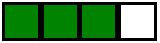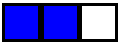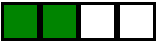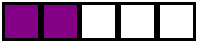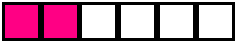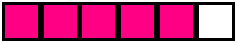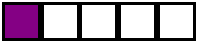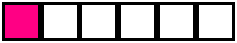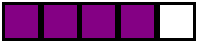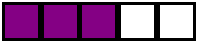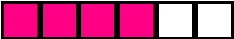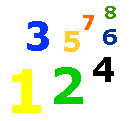# Fractions

Fraction Lesson - Numerator Lesson - Denominator Lesson - My Schoolhouse - Online Learning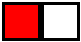A fraction is a part of a whole.  1/2 is a fraction.  Look at the rectangle above.  It is divided into two equal parts.  It is divided in half.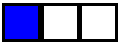Look at the rectangle above.  It is divided into 3 parts.   The blue section is 1/3 of the whole.  Each part of the rectangle is 1/3 of the whole.  There are three 1/3's in the whole rectangle.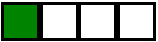The green section is 1/4 of the whole rectangle.  There are 4 parts to the whole.  Each part is 1/4 of the whole rectangle.

What is the fraction for each rectangle below?